Courses

# Moment of a Force (Vector Formulation) Mechanical Engineering Notes | EduRev

## Mechanical Engineering : Moment of a Force (Vector Formulation) Mechanical Engineering Notes | EduRev

The document Moment of a Force (Vector Formulation) Mechanical Engineering Notes | EduRev is a part of the Mechanical Engineering Course Engineering Mechanics - Notes, Videos, MCQs & PPTs.
All you need of Mechanical Engineering at this link: Mechanical Engineering

Moment of a force: vector Formulation

The cross product:

Moment of Force F around point O:MO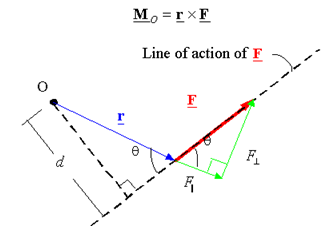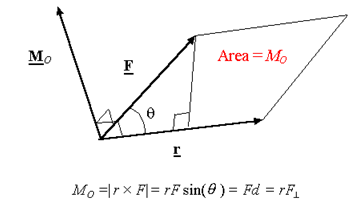Calculating the moment using rectangular components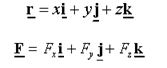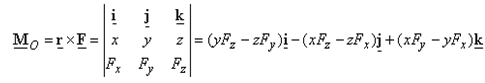Resultant moment:Mro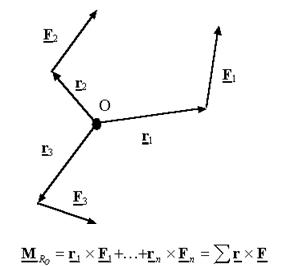Moment of a force about a specified axis a-a:Ma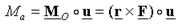O: any point on a-a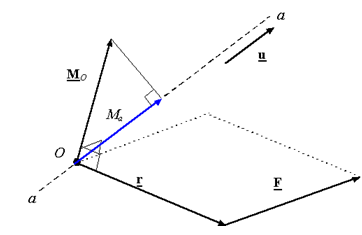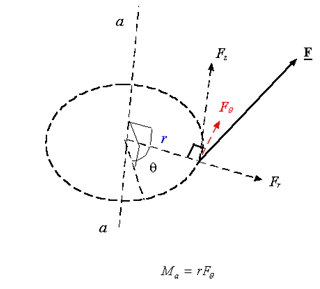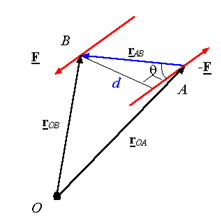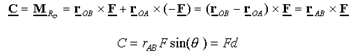Note:The moment of a couple does not depend on the point one takes the moment about. In other words, a moment of a couple is the same about all points in space.

Offer running on EduRev: Apply code STAYHOME200 to get INR 200 off on our premium plan EduRev Infinity!

,

,

,

,

,

,

,

,

,

,

,

,

,

,

,

,

,

,

,

,

,

;